Maths-
General
Easy

Question

# How can you use polynomial identities to factor polynomials and simplify numerical expressions ?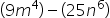Hint:

## The correct answer is: (3m2 - 5n3)(3m2 + 5n3)

### Step 1 of 2:Here, the given expression is.It can be written as: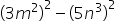, where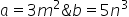.Step 2 of 2:Apply the polynomial identity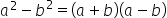to factorize the expression.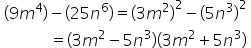Hence, the answer is: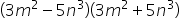Factorization of polynomial of higher degree can be done by polynomial identities. This is used to reduce time and space.#### With Turito Foundation.#### Get an Expert Advice From Turito.